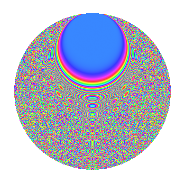# Properties

 Label 1050.2.bsLevel 1050 Weight 2 Character orbit bs Rep. character $$\chi_{1050}(23,\cdot)$$ Character field $$\Q(\zeta_{60})$$ Dimension 1280 Sturm bound 480

# Related objects

## Defining parameters

 Level: $$N$$ = $$1050 = 2 \cdot 3 \cdot 5^{2} \cdot 7$$ Weight: $$k$$ = $$2$$ Character orbit: $$[\chi]$$ = 1050.bs (of order $$60$$ and degree $$16$$) Character conductor: $$\operatorname{cond}(\chi)$$ = $$525$$ Character field: $$\Q(\zeta_{60})$$ Sturm bound: $$480$$

## Dimensions

The following table gives the dimensions of various subspaces of $$M_{2}(1050, [\chi])$$.

Total New Old
Modular forms 3968 1280 2688
Cusp forms 3712 1280 2432
Eisenstein series 256 0 256

## Trace form

 $$1280q - 4q^{7} + O(q^{10})$$ $$1280q - 4q^{7} - 4q^{10} + 24q^{15} - 160q^{16} + 8q^{18} - 56q^{22} + 8q^{25} + 72q^{27} + 16q^{28} + 12q^{30} + 20q^{33} + 8q^{37} + 40q^{39} + 40q^{42} + 64q^{43} - 52q^{45} + 16q^{55} + 136q^{57} + 28q^{58} - 8q^{60} - 12q^{63} + 16q^{67} - 280q^{69} - 68q^{70} - 8q^{72} + 56q^{73} + 152q^{75} + 16q^{78} + 64q^{82} - 60q^{84} - 32q^{85} - 76q^{87} - 12q^{88} - 160q^{90} - 84q^{93} - 64q^{97} + O(q^{100})$$

## Decomposition of $$S_{2}^{\mathrm{new}}(1050, [\chi])$$ into newform subspaces

The newforms in this space have not yet been added to the LMFDB.

## Decomposition of $$S_{2}^{\mathrm{old}}(1050, [\chi])$$ into lower level spaces

$$S_{2}^{\mathrm{old}}(1050, [\chi]) \cong$$ $$S_{2}^{\mathrm{new}}(525, [\chi])$$$$^{\oplus 2}$$

## Hecke Characteristic Polynomials

There are no characteristic polynomials of Hecke operators in the database